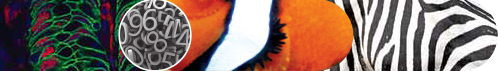# Mathematical Explanation for Changing Patterns in Nature

Spots and stripes found in animals; moles and spots on human skin; can they be explained in mathematical terms? The answer is definitely yes. Not only that, mathematics is the tool for everything from the daily budget for grocery to cosmic phenomena. The most frequently used model to explain patterns in nature like spots on deer and stripes on zebra are partial differential equations for the reaction-diffusion systems. Partial differential equations come in handy because a differential operator is needed to express the relationship of a variable with time and space. While simple physical phenomena can be easily explained by relatively simple mathematical equations, phenomena that involve more variables would require complex reaction-diffusion equations.

Complex mathematical models for complex phenomena

Prof. Wei Juncheng, recipient of the Croucher Senior Research Fellowship and Morningside Silver Medal in Mathematics, is one of the leading figures in the field of partial differential equations, in particular, the analysis of concentration phenomena in nonlinear elliptic equations and systems. His work was published in leading mathematical journals and he is amongst the 20 most-cited authors in his field. One of Professor Wei’s major discoveries was finding a counter example related to the de Giorgi Conjecture.

The de Giorgi Conjecture is one of the most famous conjectures in pure mathematics developed in 1978. It concerns the structure of certain nonlinear equations. As it was shown to be true in the second to the eighth dimensions, mathematicians since then have always held that the conjecture would apply in any number of dimensions. In 2006, through an ingenious mathematical method, Professor Wei’s team found a counter example in the ninth dimension and show that the de Giogi Conjecture could not have applied in any dimension higher than the eighth. This finding also helped solve the complex reaction-diffusion equations. It was found that with the increase of the diffusion parameter in the equations, the spots found in nature would become unstable and might split into two or even more to form complex patterns. What seem to be random and haphazard patterns in nature can actually be deciphered by mathematical models.

Practical applications of mathematical models

To further strengthen the research in nonlinear partial differential equations and nonlinear analysis, Professor Wei collaborated with the Chinese Academy of Sciences (CAS) and established the CAS-Croucher Joint Laboratory (the Lab) on “Nonlinear Partial Differential Equations and Nonlinear Analysis”. There are two missions of the Lab. The first mission is to provide top-level scientific training of PhD students and young researchers in these fields. The second is to conduct research beyond theoretical advances in Nonlinear PDEs/Nonlinear Analysis. The Lab will encourage practical applications of Nonlinear PDEs/Nonlinear Analysis in mathematical biology, fluid mechanics, material sciences, etc. It is the vision of Professor Wei and his collaborators at CAS that this will become the center for PDEs/Analysis in China as well as in the world. It will provide mathematical training and research that will complement the work of the Institute of Mathematical Sciences at CUHK and the National Interdisciplinary Science Center at CAS.

In recognition of his contributions, the International Congress of Mathematicians (ICM) has invited Professor Wei to give a 45-minute talk at its 27th congress to be held in Seoul in 2014. This is a rare and most prestigious honour for a mathematician as ICM is held every four years, an occasion considered the Olympics of the Mathematicians.